想出一道不错的面试题，有谁能做出来？ 热

ProgramFarmer 发布于 2016/06/27 11:32

【福利】CTO直接拍板说好用的SDK全在这里了！0元配齐，全部免费！>>>现在已经进入大数据时代，大数据分析一个很重要的应用就是推荐系统。假设在一个系统内，规定两个用户有两个以上相同的关注或感兴趣物品，就把这两个用户视为兴趣相同的人。比如，用户a关注了物品1、2、3，用户b关注了物品2、3、4，用户a和b都关注了物品2、3，a和b算兴趣相同的人，则把用户b关注的物品4推荐给用户a。

完成下面代码中computeRecommend方法的功能，要求尽可能高效。

public static Map<String, int[]> computeRecommend(Map<String, int[]> data) {
// todo
return null;
}

public static void main(String[] args) {
String user1 = "a";
String user2 = "b";
String user3 = "c";
Map<String, int[]> map = new HashMap<String, int[]>();
map.put(user1, new int[] { 1, 2, 3 });
map.put(user2, new int[] { 2, 3, 4, 5 });
map.put(user3, new int[] { 4, 5, 6, 7 });
Map<String, int[]> recommendMap = computeRecommend(map);
printData(recommendMap);
// expect:
// a -> (4,5)
// b -> (1,6,7)
// c -> (2,3)
}

public static void printData(Map<String, int[]> data) {
if (data == null){
System.out.println("null");
return;
}
for (Entry<String, int[]> entry : data.entrySet()) {
System.out.println(entry.getKey() + " -> " + showArr(entry.getValue()));
}
}

public static String showArr(int[] arr) {
if (arr == null)
return "null";
else {
int len = arr.length;
StringBuilder sb = new StringBuilder("(");
for (int i = 0; i < len - 1; i++)
sb.append(arr[i] + ",");
if (len > 0)
sb.append(arr[len - 1]);
sb.append(")");
return sb.toString();
}
}

以下是话题补充：

@ProgramFarmer：提示验证码不正确，怎么发重了？想删掉没权限。 (2016/06/27 11:38)

1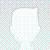import org.apache.commons.collections4.bag.HashBag;

import java.util.*;

/**
* Created by rmiao on 6/26/2016.
*/
public class Tests {

private static double FLAG=0.5;

public static void main(String[] args) {
String user1 = "a";
String user2 = "b";
String user3 = "c";
Map<String, int[]> map = new HashMap<>();
map.put(user1, new int[] { 1, 2, 3 });
map.put(user2, new int[] { 2, 3, 4, 5 });
map.put(user3, new int[] { 4, 5, 6, 7 });
Map<String, List> recommendMap = computeRecommend(map);
printData(recommendMap);
// expect:
// a -> (4,5)
// b -> (1,6,7)
// c -> (2,3)
}

public static Map<String, List> computeRecommend(Map<String, int[]> data) {

Map<String,List> recommed = new HashMap<>();
String[] nameList = initRecommendResult(data, recommed);

for (int i = 0; i < nameList.length; i++) {

for (int j = i+1; j < nameList.length; j++) {

Map<String, Object> repeatResult = getRepeatCount(data.get(nameList[i]), data.get(nameList[j]));

Set repeatSet = (Set)repeatResult.get("repeatSet");
if (repeatSet.size()>0){

adviseByPercentage(recommed, nameList[i], nameList[j], repeatResult);

}
}
}

return recommed;
}

public static Map<String, Object> getRepeatCount(int[] M, int[] N) {

Map<String,Object> result = new HashMap<>();

if (M.length==0 || N.length==0){
return result;
}

Set<Integer> setM = getUniqueSet(M);
Set<Integer> setN = getUniqueSet(N);
HashBag bag=new HashBag();

Set set = bag.uniqueSet();

Set repeatSet = new HashSet();

for (Object o : set) {
if(bag.getCount(o)>=2){
}
}

result.put("repeatSet",repeatSet);

Map<String, Object> resultM= new HashMap<>();
Map<String, Object> resultN= new HashMap<>();
setM.removeAll(repeatSet);
setN.removeAll(repeatSet);

resultM.put("percentage",repeatSet.size()/(double)setM.size());
resultM.put("unRepeatSet",setM);

resultN.put("percentage",repeatSet.size()/(double)setN.size());
resultN.put("unRepeatSet",setN);

result.put("M",resultM);
result.put("N",resultN);
return result;
}

private static Set<Integer> getUniqueSet(int[] M) {
Set<Integer> setM=new HashSet<Integer>();
for(int m:M)
return setM;
}

private static void adviseByPercentage(Map<String, List> recommed, String i, String j, Map<String, Object> repeatResult) {
HashMap m = (HashMap)repeatResult.get("M");
HashMap n = (HashMap)repeatResult.get("N");

if((double)m.get("percentage")>= FLAG){
}
if((double)n.get("percentage")>=FLAG){
}
}

private static String[] initRecommendResult(Map<String, int[]> data, Map<String, List> recommed) {
Set<String> names = data.keySet();
String[] nameList = new String[names.size()];

int index=0;
for (String s : names) {
recommed.put(s,new ArrayList());
nameList[index] = s;
index++;
}
return nameList;
}

public static void printData(Map<String, List> data) {
if (data == null){
System.out.println("null");
return;
}
for (Map.Entry<String, List> entry : data.entrySet()) {
System.out.println(entry.getKey()+" -> " + entry.getValue());
}
}

}

引言

相似度计算用于衡量对象之间的相似程度，在数据挖掘、自然语言处理中是一个基础性计算。其中的关键技术主要是两个部分，对象的特征表示，特征集合之间的相似关系。在信息检索、网页判重、推荐系统等，都涉及到对象之间或者对象和对象集合的相似性的计算。而针对不同的应用场景，受限于数据规模、时空开销等的限制，相似度计算方法的选择又会有所区别和不同。下面章节会针对不同特点的应用，进行一些常用的相似度计算方法进行介绍。

3 基于hash方法的相似计算

基于hash的相似度计算方法，是一种基于概率的高维度数据的维度削减的方法，主要用于大规模数据的压缩与实时或者快速的计算场景下，基于hash方法的相似度计算经常用于高维度大数据量的情况下，将利用原始信息不可存储与计算的问题转化为映射空间的可存储计算问题，在海量文本重复性判断方面，近似文本查询方面有比较多的应用，google的网页去重，google news的协同过滤[2,3]等都是采用hash方法进行近似相似度的计算，比较常见的应用场景Near-duplicate detection、Image similarity identification、nearest neighbor search，常用的一些方法包括I-match，Shingling、Locality-Sensitive Hashing族等方法，下面针对几种常见的hash方法进行介绍。

3.1 minhash方法介绍

Minhash方法是Locality-sensitive hashing[4,5]算法族里的一个常用方法，基本的思想是，对于每一个对象的itemlist，将输入的item进行hash，这样相似的item具有很高的相似度被映射到相同的buckets里面，这样尽量保证了hash之后两个对象之间的相似程度和原来是高相似的，而buckets的数量是远远小于输入的item的，因此又达到降低复杂度的目的。

minhash方法用Jaccard进行相似度的计算方法，则对于两个集合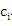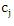，和的相似性的计算方法为：

3.2 simhash方法介绍

simhash方法是在大文本重复识别常用的一个方法，该方法主要是通过将对象的原始特征集合映射为一个固定长度的签名，将对象之间的相似度的度量转化为签名的汉明距离，通过这样的方式，极大限度地进行了降低了计算和存储的消耗。

3.2.1 签名计算过程

该方法通过对输入特征集合的计算步骤可以描述如下：

1. 将一个f维的向量V初始化为0；f位的二进制数S初始化为0；
2. 对每一个特征：用传统的hash算法对该特征产生一个f位的签名b。对i=1到f：

如果b的第i位为1，则V的第i个元素加上该特征的权重；

1. 如果V的第i个元素大于0，则S的第i位为1，否则为0；
2. 输出S作为签名。

3.3 小结

hash方法的相似度计算的主要应用场景，一般是针对大规模数据进行压缩，在保证效果损失可接受的情况下，节省存储空间，加快运算速度，针对该方法的应用，在目前的大规模的互联网处理中，很多相似度的计算都是基于这种近似性的计算，并取得了比较好的效果。

4 基于主题的相似度计算

传统的BOW（bag-of_words）模型，一般都会建立在特征独立假设的基础上，按照特征向量的匹配情况来度量对象之间的相似度，但是在实际的应用中，很多时候特征之间存在着很多的关联关系，二者在传统的BOW模型中无法解决，在这个基础上，引入了主题的概念，通过主题的思想，建立起基本特征与对象的中间层的关联关系，主题的概念的引入，主要是在原有的基本特征粒度的基础上，引入了更为丰富的隐含层特征，提高了相似性计算的效果，常用的主题分析方法包括Latent Semantic Analysis (LSA) 、 Probabilitistic Latent Semantic Analysis (PLSA)、Latent Dirichlet Allocation ( LDA)。这些方法在分类，聚类、检索、推荐等领域都有着很多的应用，并取得了比较好的效果。下面就LSA及PLSA方法进行简要介绍。

4.1 LSA简介

LSA[6,7]模型认为特征之间存在某种潜在的关联结构，通过特征-对象矩阵进行统计计算，将高维空间映射到低纬的潜在语义结构上，构建出LSA空间模型，从而提取出潜在的语义结构，并用该结构表示特征和对象，消除了词汇之间的相关性影响，并降低了数据维度。增强了特征的鲁棒性

LSA利用奇异值分解来进行计算，数学过程可以表述如下：

对于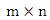的矩阵A，其中m为特征数，n为样本数。令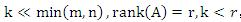，经过奇异值分解，矩阵A可分解成3个矩阵的乘积：

4.2 plas介绍

PLSA[8,9]模型是由Hofmann提出的用于文本检索的概率生成模型，与相比较于LSA，PLSA是基于概率模型的，并直接引入了潜在class变量 ，下面的用文本处理语言来描述该模型。

(7)

(8)

(9)

PLSA和其相关的变形，在分类、聚类、检索等方面，特征相关性计算等方面，获得了广泛的应用，并取得了比较好的效果。

4.2 plas介绍

PLSA[8,9]模型是由Hofmann提出的用于文本检索的概率生成模型，与相比较于LSA，PLSA是基于概率模型的，并直接引入了潜在class变量 z∈Z=｛Z1…Zk ｝，下面的用文本处理语言来描述该模型。

PLSA和其相关的变形，在分类、聚类、检索等方面，特征相关性计算等方面，获得了广泛的应用，并取得了比较好的效果。

.3 小结

主题方法的引入，在一定程度上弥补了BOW的假设的独立性，在工业中，主题的方法也越来越多的应用到实际的机器学习中，包括在图像处理领域、传统的分类、聚类、检索等方面，都取得了比较好的效果。

总结

相似度的计算在数据挖掘方面有着广泛的应用，根据不同的应用场景，各种方法各有其优劣特点，对于相似度效果的影响，除了方法本身之外，合理有效的特征的选择和使用也是至关重要的，同时，根据应用场景的不同，选择合理的方法，对于解决问题，有着重要的作用。

1. G.S. Manku, A. Jain, A.D. Sarma. Detecting Near-Duplicates for Web Crawling. WWW2007, 2007

2. A. Das, M. Datar, A.Garg. Google News Personalization: Scalable Online Collaborative Filtering. WWW2007, 2007

4. M. S. Charikar. Similarity estimation techniques from rounding algorithms. STOC’02. 2002

6. K. Dave, S. Lawrence, and D. Pennock. Mining the peanut gallery: opinion extraction and semantic classification of product reviews. In Proceedings of the 22th International World Wide Web Conference, Budapest, Hungary, 2003

8. T. Hofmann. Probabilistic Latent Semantic Analysis. In Proceedings of the 15th Conference on Uncertainty in AI(1999).

9. Y. M kim, J. F. Pressiot M. R.Amini etc. An Extension of PLSA for Document Clustering. CIKM’080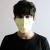0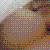0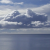public static Map<String, int[]> computeRecommend(Map<String, int[]> data) {
int size = data.size();

Map<String, Set<Integer>> recommends = new HashMap<>(size);// 存放推荐的数据

List<Entry<String, int[]>> entryList = new ArrayList<>(size);// 原数据转换为List处理
for (Entry<String, int[]> entry : data.entrySet()) {
recommends.put(entry.getKey(), new HashSet<Integer>());
}
for (int i = 0; i < size - 1; i++) {
for (int j = i + 1; j < size; j++) {
int[] arr1 = entryList.get(i).getValue();
int[] arr2 = entryList.get(j).getValue();
Set<Integer> commons = compareArr(arr1, arr2);
if (commons.size() > 1) {
swapInterest(arr1, recommends.get(entryList.get(i).getKey()), //交换
arr2, recommends.get(entryList.get(j).getKey()), commons);
}
}
}

//转换为结果要的形式
Map<String, int[]> result = new HashMap<>(size);
for (Entry<String, Set<Integer>> entry : recommends.entrySet()) {
result.put(entry.getKey(), setToArr(entry.getValue()));
}
return result;
}

public static Set<Integer> compareArr(int[] arr1, int[] arr2) {
Set<Integer> commons = new HashSet<>();
for (int i = 0, len1 = arr1.length; i < len1; i++) {
for (int j = 0, len2 = arr2.length; j < len2; j++) {
if (arr1[i] == arr2[j])
}
}
return commons;
}

public static void swapInterest(int[] arr1, Set<Integer> set1, int[] arr2, Set<Integer> set2,
Set<Integer> commons) {
for (int x : arr2) {
if (!commons.contains(x))
}
for (int y : arr1) {
if (!commons.contains(y))
}
}

public static int[] setToArr(Set<Integer> set) {
int len = set.size();
List<Integer> list = new ArrayList<Integer>(set);
int[] arr = new int[len];
for (int i = 0; i < len; i++)
arr[i] = list.get(i);
return arr;
}

0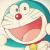@Test
public void test(){
String user1 = "a";
String user2 = "b";
String user3 = "c";
Map<String, int[]> map = new HashMap<String, int[]>();
map.put(user1, new int[] {1, 2, 3});
map.put(user2, new int[] {2, 3, 4, 5});
map.put(user3, new int[] {4, 5, 6, 7});

Map<String, int[]> recommends = new  HashMap<String, int[]>();

for(Iterator<String> it = map.keySet().iterator(); it.hasNext();){
String k1 = it.next();
for(String k2 : map.keySet()){
if(k1.equals(k2)){
continue;
}
int[] hobby1 = map.get(k1), hobby2 = map.get(k2);
int[][] recommend = isRecommend(hobby1, hobby2);
if(recommend == null){
continue;
}

if(recommends.containsKey(k1)){
recommends.put(k1, merge(recommends.get(k1), recommend));
} else {
recommends.put(k1, recommend);
}
if(recommends.containsKey(k2)){
recommends.put(k2, merge(recommends.get(k2), recommend));
} else {
recommends.put(k2, recommend);
}
}
it.remove();
}
printData(recommends);
}

private void printData(Map<String, int[]> data) {
if (data == null){
System.out.println("null");
return;
}
for (Entry<String, int[]> entry : data.entrySet()) {
System.out.println(entry.getKey() + " -> " + Arrays.toString(entry.getValue()));
}
}

private int[][] isRecommend(int[] ary1, int[] ary2){
int[] merge = merge(ary1, ary2);
if(merge.length == ary1.length + ary2.length){
return null;
}
return new int[][]{findRecommend(merge, ary1), findRecommend(merge, ary2)};
}

private int[] findRecommend(int[] merge, int[] ary){
int[] r = new int[merge.length - ary.length];
for(int i = 0, j = 0; j < merge.length && i < r.length; j++){
if(!isContains(merge[j], ary)){
r[i++] = merge[j];
}
}
return r;
}

private boolean isContains(int i, int[] ary){
for(int v : ary){
if(i == v) return true;
}
return false;
}

private int[] merge(int[] ary1, int[] ary2){
int[] merge = new int[ary1.length + ary2.length];
int count = 0;
for(int i = 0, j = 0, k = 0; k < merge.length; k++,count++){
if(i == ary1.length && j == ary2.length){
break;
}
if(i < ary1.length && j < ary2.length){
if(ary1[i] == ary2[j]){
merge[k] = ary1[i++];
j++;
continue;
}
if(ary1[i] < ary2[j] ){
merge[k] = ary1[i++];
} else {
merge[k] = ary2[j++];
}
}
if(i == ary1.length && j < ary2.length){
merge[k] = ary2[j++];
}
if(i < ary1.length && j == ary2.length){
merge[k] = ary1[i++];
}
}
return Arrays.copyOf(merge, count);
}

0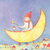import java.util.ArrayList;
import java.util.BitSet;
import java.util.HashMap;
import java.util.List;
import java.util.Map;

public class Test {

public static void main(String[] args) {

String user1 = "a";
String user2 = "b";
String user3 = "c";
String user4 = "d";
Map<String, int[]> map = new HashMap<String, int[]>();
map.put(user1, new int[] { 1, 2, 3 });
map.put(user2, new int[] { 2, 3, 4, 5 });
map.put(user3, new int[] { 4, 5, 6, 7 });
map.put(user4, new int[] { 2, 3, 6, 7, 11, 21 });
Map<String, BitSet> maps = new HashMap<String, BitSet>();

List<BitSet> lists = new ArrayList<>();
for (String user : map.keySet()) {
int[] ints = map.get(user);
BitSet bits = new BitSet();
for (int i : ints) {
bits.set(i);
}
System.out.println(user + "-->" + bits);
maps.put(user, bits);
}
Map<String, BitSet> retMaps = new HashMap<String, BitSet>();
System.out.println("\n--------------操作----------------\n");
for (String user : maps.keySet()) {
BitSet _newBits = new BitSet();
BitSet baseBits = maps.get(user);

lists.stream().filter(t -> !t.equals(baseBits)).forEach(t -> {
BitSet cloneBits = (BitSet) t.clone();
cloneBits.and(baseBits);
if (cloneBits.cardinality() >= 2) {
BitSet b = ((BitSet) t.clone());
b.andNot(cloneBits);
_newBits.or(b);
}
});

retMaps.put(user, _newBits);
}

for (String user : retMaps.keySet()) {
System.out.println(user + "-->" + retMaps.get(user));
}
}

}